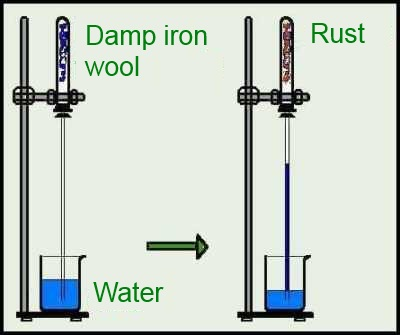Search:

# The (slow) oxidation of iron

## Formation of rustPart of the air initially present in the tube is consumed: It is the oxygen. It reacts with iron to produce rust: $2Fe$ $+$ $3O_2$ $\longrightarrow$ $2Fe_2O_3$ The water is needed to hydrate the iron oxide: $Fe_2O_3$ $+$ $3H_2O$ $\longrightarrow$ $Fe_2O_3\cdot3H_2O(rust)$